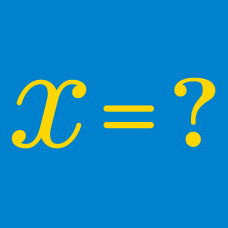Algebra

# Negative Exponents - Numerical

Find the value of $3^{-3}$.

What is the value of $(-4)^{-2}$?

What is the value of the following expression:

$5^{-1} \times 2^3 \times 3^{-2} ?$

Scientific notation has two parts: a number (say $a$) between one and ten, and that number multiplied by ten raised to the power $b,$ where $b$ is a non-zero integer: e.g. $a \times 10^{b},$ where $1 \le a<10$.

Find the value of $a$ when you simplify the following expression in scientific notation to two decimal points:

$(4.7 \times 10^{-3}) \times (6.1 \times 10^{9}).$

Find the value of $\left( \frac{1}{4} \right)^{-2}$.

×